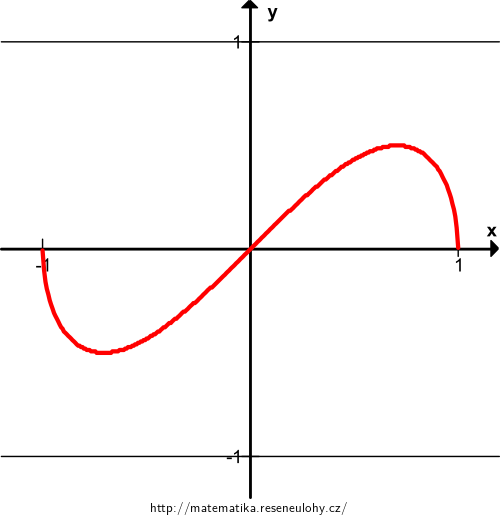## Shape of a function

Investigate the shape of the function $$f(x)=x\sqrt{1-x^2}$$.

That is, determine its domain of definition, range, extrema, inflection points, asymptotes; investigate monotonicity, convexity, concavity, behavior at boundary points of the domain of definition. With these characteristics in mind, sketch its graph.

• #### Resolution

The function is odd and continuous.

The domain of definition $$D_f=\langle -1, 1\rangle$$.

$$f(x)=0$$ for $$x\in\{-1{,}0,1\}$$.

$$\displaystyle f'(x)= 1\cdot \sqrt{1-x^2}+ x \cdot \frac1{2\sqrt{1-x^2}}\cdot (-2x)= \sqrt{1-x^2}- \frac{x^2}{\sqrt{1-x^2}}$$

$$f'(x)=0$$ for $$x\in\{\frac{-\sqrt2}2,\frac{\sqrt2}2\}$$.

On $$\langle-1,\frac{-\sqrt2}2\rangle\cup\langle\frac{\sqrt2}2{,}1\rangle$$ the function is decreasing, on $$\langle\frac{-\sqrt2}2,\frac{\sqrt2}2\rangle$$ it is increasing.

$$f\left(\frac{-\sqrt2}2\right)=-\frac12$$ is a minimum, and $$f\left(\frac{\sqrt2}2\right)=\frac12$$ is a maximum. The range $$H_f=\left\langle-\frac12,\frac12\right\rangle$$.

$$\displaystyle f''(x)= \frac1{2\sqrt{1-x^2}}\cdot (-2x) -\frac{2x}{\sqrt{1-x^2}} + \frac{x^2\cdot(-2x)}{2\sqrt{1-x^2}^{3}}= -\frac{2x}{\sqrt{1-x^2}} - \frac{x^3}{\sqrt{1-x^2}^{3}}$$

$$f''(x)=0$$ for $$x=0$$.

On $$\langle-1{,}0\rangle$$ the function is convex; on $$\langle0{,}1\rangle$$ it is concave. 0 is an inflection point.

$$\displaystyle \lim_{x\to 0} f(x)=1,\ \lim_{x\to 1^-} f(x)=-\infty,\ \lim_{x\to -1^+} f(x)=-\infty$$.